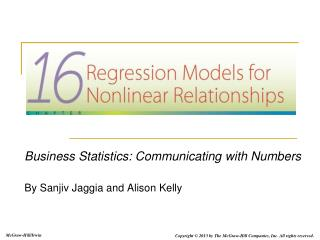# Business Statistics: Communicating with Numbers By Sanjiv Jaggia and Alison Kelly - PowerPoint PPT PresentationDownload PresentationBusiness Statistics: Communicating with Numbers By Sanjiv Jaggia and Alison Kelly

Business Statistics: Communicating with Numbers By Sanjiv Jaggia and Alison KellyDownload Presentation## Business Statistics: Communicating with Numbers By Sanjiv Jaggia and Alison Kelly

- - - - - - - - - - - - - - - - - - - - - - - - - - - E N D - - - - - - - - - - - - - - - - - - - - - - - - - - -
##### Presentation Transcript

1. Business Statistics: Communicating with Numbers By Sanjiv Jaggia and Alison Kelly

2. Chapter 16 Learning Objectives (LOs) LO 16.1:Use and evaluate polynomial regression models. LO 16.2:Use and evaluate log-transformed models. LO 16.3:Describe the method used to compare linear with log-transformed models.

3. The Rental Market in Ann Arbor, Michigan • Marcela Treisman is analyzing the rental market in Ann Arbor, the home of the University of Michigan. • She has data on monthly rent, along with several property characteristics. • Marcela will evaluate models that predict rental income from home characteristics. She will select the most appropriate model and make predictions for rental income for specific property characteristics. 16-3

4. 16.1Polynomial Regression Models LO 16.1 Use and evaluate polynomial regression models. 16-4

5. Example 16.1 LO 16.1 • Suppose we want to estimate the relationship between average cost and output. We gather data for 20 manufacturing firms on output and average cost. • When using a scatterplot to display the relationship, notice that a quadratic curve seems to better fit the data. 16-7

6. Example 16.1 LO 16.1 • We then estimate both a linear and quadratic model, determining which is more appropriate from our goodness-of-fit measures. • In order to estimate a quadratic regression model, we first need to compute the squared output: 16-8

7. Estimation Results LO 16.1 16-9

8. The Marginal Effect LO 16.1 16-10

9. Maximum or Minimum? LO 16.1 16-11

10. Example 16.2 LO 16.1 16-12

11. Higher Order Models LO 16.1 16-13

12. Example 16.4 LO 16.1 • We often use a cubic model to estimate a firm’s total costs. • Let’s work through an example using the data labeled Total Cost on the text website. Here we are given the total cost for various levels of output. • We first must compute output squared and output cubed before estimating the model: 16-14

13. Example 16.4 LO 16.1 16-15

14. 16.2 Regression Models with Logarithms LO 16.2 Use and evaluate log-transformed models.

15. The Log-Log Model LO 16.2

16. The Slope as an Elasticity LO 16.2

17. Prediction with the Model LO 16.2

18. Example 16.5 LO 16.2

19. The Logarithmic Model LO 16.2 16-21

20. Example 16.6 LO 16.2

21. The Exponential Model LO 16.2 16-24

22. Example 16.7 LO 16.2

23. Summary of Logarithmic Models LO 16.2 16-27

24. Ann Arbor Rentals LO 16.2 • In the introductory case, Marcela Treisman wants to examine what factors influence rental prices in Ann Arbor, Michigan. • She has data on 40 properties, including the number of bedrooms and baths, the floor space, and the rental price. • As a first look, she plots each of the explanatory variables against the rental price.

25. Plotting the Data LO 16.2 • When plotting the number of bedrooms and number of bathrooms against Rent, the relationship appears to be roughly linear.

26. Plotting the Data LO 16.2 • Since the relationship between rent and floor space seems to change as the floor space increases, we should consider possible logarithmic models. 16-30

27. Potential Models LO 16.2

28. Estimation Results LO 16.2 • Since all three models have three explanatory variables, we need not present the adjusted R2. • Yet, we cannot simply compare the standard error and R2 of all four models since the response variable differs. 16-32

29. Predicting the Rental Price LO 16.2

30. Comparing Linear and Log-Transformed Models LO 16.3 Describe the method used to compare linear with log-transformed models.

31. Computing R2 LO 16.3

32. Ann Arbor Rentals LO 16.3 • Let’s return to model selection for the introductory case. • Recall that of the models that used an untransformed response variable, the logarithmic model performed best. • Of the models that used the transformed response variable, the log-log model outperformed the exponential model. • But we now have the tools to select the most appropriate model overall.

33. Computing R2 LO 16.3

34. Conclusion LO 16.3 16-38